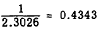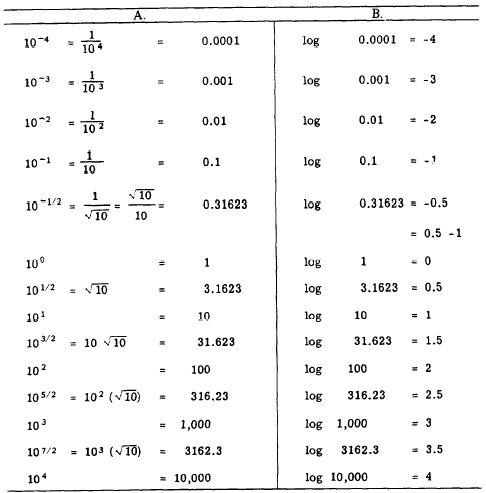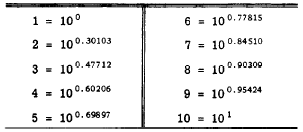Common LogarithmsCustom SearchCOMMON LOGARITHMS As has been shown in preceding paragraphs, any number may be used as a base for a system of logarithms. The selection of a base is a matter of convenience. Briggs in 1617 found that base 10 possessed many advantages not obtainable in ordinary calculations with other bases. The selection of 10 as a base proved so satisfactory that today it is used almost exclusively for ordinary calculations. Logarithms with 10 as a base are therefore called COMMON LOGARITHMS. When 10 is used as a base, it is not necessary to indicate it in writing logarithms. For example, log 100 = 2 is understood to mean the same as log 10 100 = 2 If the base is other than 10, it must be specified by the use of a subscript to the right and below the abbreviation "log." As noted in the foregoing discussion of natural logarithms, the use of the distinctive abbreviation "In" eliminates the need for a subscript when the base is e. It is relatively easy to convert common logarithms to natural logarithms or vice versa, if necessary. It should be noted further that each system has its peculiar advantages, but for most everyday work, the common system is more often used. A simple relation connects the two systems. If the common logarithm of a number can be found, multiplying by 2.3026 gives the natural logarithm of the number. For example,  log 1.60 = 0.2041     ln 1.60 = 2.3026 x 0.2041                 = 0.4700  Thus the natural logarithm of 1.60 is 0.4700, correct to four significant digits. Conversely, multiplying the natural logarithm by 0.4343 gives the common logarithm of a number. As might be expected, the conversion factor 0.4343 is the reciprocal of 2.3026. This is shown as follows:Positive Integral Logarithms The derivation of positive whole logarithms is readily apparent. For example, we see in table 8-3 (B) that the logarithm of 10 is 1. The number 1 is simply the exponent of the base 10 which yields 10. This is shown in table 8-3 (A) opposite the logarithmic equation. Similarly, 100=1 . . . . . . . . . . . log 1=0 102 = 100. . . . . . . . . log 100 = 2 103 = 1,000 . . . . . . log 1,000 = 3 104 = 10,000. . . . . log 10,000 = 4 Table 8-3.-Exponential and corresponding logarithmic notations using base 10.Positive Fractional Logarithms Referring to table 8-3, notice that the logarithm of 1 is 0 and the logarithm of 10 is 1. Therefore, the logarithm of a number between 1 and 10 is between 0 and 1. An easy way to verify this is to consider some numbers between 1 and 10 which are powers of 10; the exponent in each case will then be the logarithm we seek. Of course, the only powers of 10 which produce numbers between 1 and 10 are fractional powers. EXAMPLE: 101/2 = 3.1623 (approximately)                       100.5 = 3.1623 Therefore, log     3.1623 = 0.5 Other examples are shown in the table for 103/2 , 105/2, and 107/2. Notice that the number that represents 103/2 , 31.623, logically enough lies between the numbers representing 101 and 102 -that is, between 10 and 100. Notice also that 105/2 appears between 102 and 103, and 107/2 lies between lo3 and 104. Negative Logarithms Table 8-3 shows that negative powers of 10 may be fitted into the system of logarithms. We recall that 10-1 means 1/10, or the decimal fraction, 0.1. What is the logarithm of 0.1? SOLUTION: 10-1 = 0.1; log 0.1 = -1 Likewise 10-2 = 0.01; log 0.01 = -2 Negative Fractional Logarithms Notice in table 8-3 that negative fractional exponents present no new problem in logarithmic notation. For example, 1O’1’2 meansWhat is the logarithm of 0.316231 SOLUTION: 10-1/2 = 0.31623; log 0.31623 = -1/2                                                     = -0.5 Table 8-3 shows logarithms for numbers ranging from 0.0001 to 10,000. Notice that there are only 8 integral logarithms in the entire range. Excluding zero logarithms, the logarithms for all other numbers in the range are fractional or contain a fractional part. By the year 1628, logarithms for all integers from 1 to 100,000 had been computed. Practically all of these logarithms contain a fractional part. It should be remembered that finding the logarithm of a number is nothing more than expressing the number as a power of 10. Table 8-4 shows the numbers 1 through 10 expressed as powers of 10. Most of the exponents which comprise logarithms are found by methods be- yond the scope of this text. However, it is not necessary to know the process used to obtain logarithms in order to make use of them. Table 8-4.-The numbers 1 through 10 expressed as powers of 10.Integrated Publishing, Inc. - A (SDVOSB) Service Disabled Veteran Owned Small Business